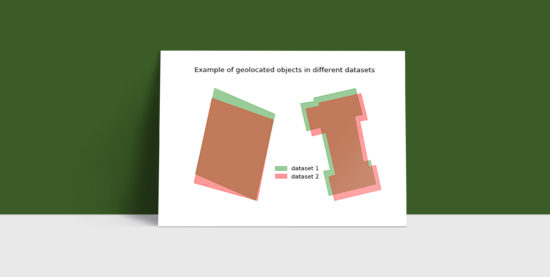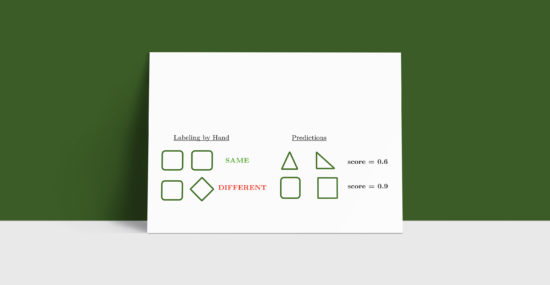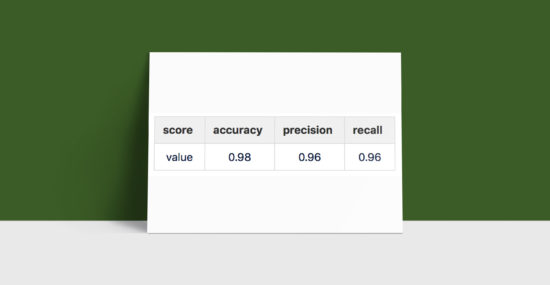+33 1 85 800 801 contact@namr.com

# Merging geospatial data

Today we are going to discuss on geospatial data. And to build a rich description of the object, the real challenge is to join these pieces together. But in absence of a clear and coherent name or index, this process can become quite difficult and noisy.For example when trying to aggregate information on a building we may find its price per square meter in a file, while another file may contain information on its heating system or material. Of course though buildings usually don’t have names, and are often indexed differently according to the source, or institution, behind the creation of the dataset. This means that to correctly merge the various sources one has to be creative.

Luckily more often than not the geographical objects come with the coordinates that describe their geometry, and this indeed can be used to merge the data on twin polygons. By twin polygons we mean polygons describing the same object in the different datasets.

That said one can be surprised to see how many small differences are found in the coordinates of one same object coming from different sources: the angles, the number of vertexes, the length of the edges and of course the geolocalization are often slightly different. Moreover a single building in a dataset can be easily represented as several buildings in another one. In the figure above we can see two tricky examples. All this implies that a unique definition of twin polygons does not exist and therefore we leave this task to an algorithm.

### Machine Learning Approach: A Shape Matching AlgorithmThe Machine Learning approach we chose consists of training a Random Forest algorithm to give a similarity score of two polygons. To do this we started by describing polygons through a number of geometrical features: compactness, PCA orientation, eccentricity, convexity and more. A polygon is therefore interpreted as a vector of numbers like in the figure above. This allowed us to quantify the difference of any given couple as the difference of these vectors .To train our Random Forest algorithm we labeled by hand more than 20 000 couples of polygons, coming from spatial intersections of real datasets, as same or different.

The algorithm then learnt to give a similarity score based on the geometrical features of two polygons. This can be interpreted as the probability of the two polygons being the same one. By setting a threshold at t = 0.7 on the similarity score we obtained the following performances on our training set.We considered these values good enough, and therefore started using this algorithm to put some order in the wildness of the geospatial data universe.

May the merging begin!